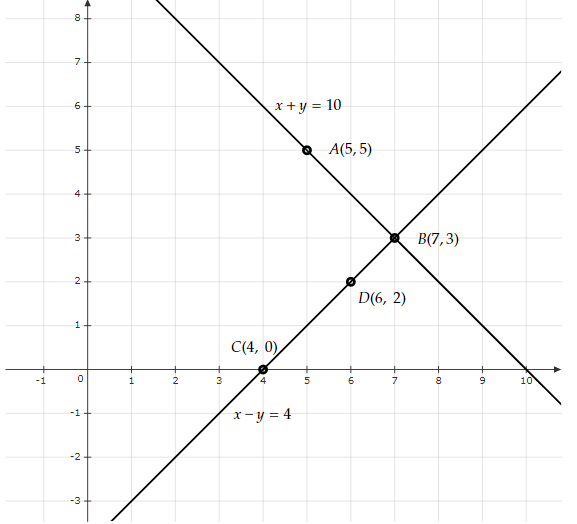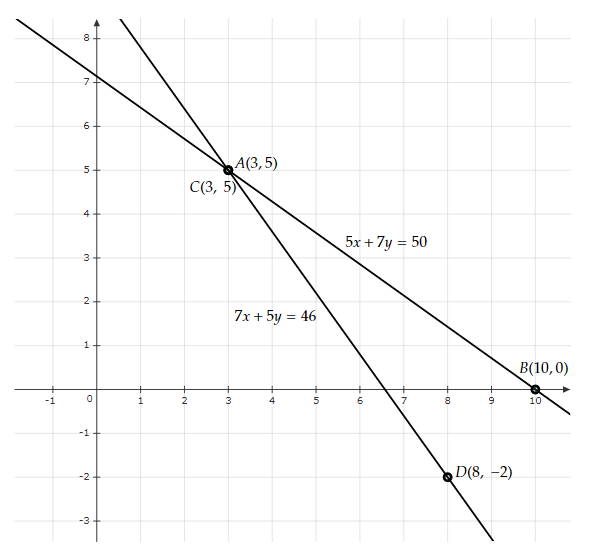# Form the pair of linear equations in the following problems, and find their solutions graphically.(i) 10 students of class X took part in a Mathematics quiz. If the number of girls is 4 more than the number of boys, find the number of boys and girls who took part in the quiz.(ii) 5 pencils and 7 pens together cost Rs. 50, whereas 7 pencils and 5 pens together cost Rs. 46. Find the cost of one pencil and that of one pen.

Given:

10 students of class X took part in the Mathematics quiz. The number of girls is 4 more than the number of boys.

To do:

We have to form a pair of linear equations and solve them graphically. Also, we have to find the number of boys and girls who took part in the quiz.

Solution:

(i) Let the number of girls and boys in the class be $x$ and $y$ respectively.

According to the question,

$x + y = 10$.....(i)

$x - y = 4$.....(ii)

To represent the above equations graphically we need at least two solutions for each of the equations.

For equation $x+y=10$,

$y=10-x$

If $x=5$ then $y=10-5=5$

If $x=7$ then $y=10-7=3$

 $x$ $5$ $7$ $y$ $5$ $3$

For equation $x-y=4$,

$y=x-4$

If $x=4$ then $y=4-4=0$

If $x=6$ then $y=6-4=2$

 $x$ $4$ $6$ $y$ $0$ $2$

The above situation can be plotted graphically as below:The lines AB and CD represent the equations $x+y=10$ and $x-y=4$ respectively.

The solution of the above equations is the point of intersection.

Therefore,

Number of girls $x=7$

Number of boys $y=3$

The number of boys and girls who took part in the quiz is $3$ and $7$ respectively.

(ii) Let the cost of one pencil and one pen be $x$ and $y$ respectively.

According to the question,

$5x + 7y = 50$.....(i)

$7x + 5y = 46$.....(ii)

To represent the above equations graphically we need at least two solutions for each of the equations.

For equation $5x+7y=50$,

$7y=50-5x$

$y=\frac{50-5x}{7}$

If $x=3$ then $y=\frac{50-5(3)}{7}=\frac{50-15}{7}=\frac{35}{7}=5$

If $x=10$ then $y=\frac{50-5(10)}{7}=\frac{50-50}{7}=\frac{0}{7}=0$

 $x$ $3$ $10$ $y$ $5$ $0$

For equation $7x+5y=46$,

$5y=46-7x$

$y=\frac{46-7x}{5}$

If $x=3$ then $y=\frac{46-7(3)}{5}=\frac{46-21}{5}=\frac{25}{5}=5$

If $x=8$ then $y=\frac{46-7(8)}{5}=\frac{46-56}{5}=\frac{-10}{5}=-2$

 $x$ $3$ $8$ $y$ $5$ $-2$

The above situation can be plotted graphically as below:The lines AB and CD represent the equations $5x+7y=50$ and $7x+5y=46$ respectively.

The solution of the above equations is the point of intersection.

Therefore,

Cost of one pencil $x=3$

Cost of one pen $y=5$

The cost of one pencil and a pen is $3$ and $5$ respectively.

Updated on: 10-Oct-2022

56 Views